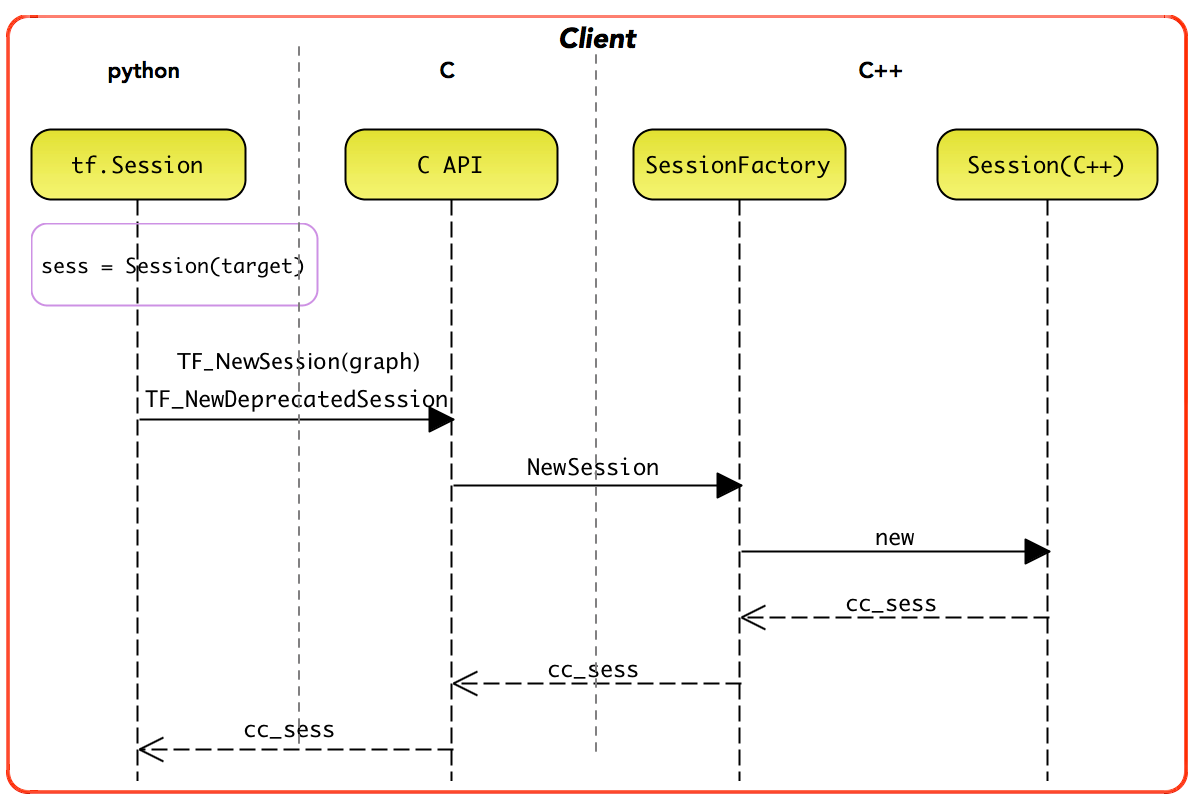2022年03月02日

## 简介

python 是解释型语言，性能是瓶颈。实现混合编程的方式

1. 使用ctypes 库加载c++编写的动态链接库
2. 使用pybind 将c++编译为python库
3. 使用pythran库 将python直接转换为C++代码

1. 一些要素 在跨语言间的对应关系，比如c++ 的对象、函数、全局变量。c++ 函数 暴露到 python 成为 一个模块下的函数，c++ 对象则暴露为 一个python 对象。 比如在jna 中，c++ 函数会被暴露为 一个接口的方法，jna 负责提供这个接口的实现。
2. 常见的类型（作为参数的时候） 如何跨语言打通，比如string、vector、map以及指针

tf 从swig 切到了pybind11。

## swig

tf 早期通过swig 实现python 调用c

1. 在 pywrap_tensorflow_internal.cc 的实现中，静 态注册了一个函数符号表，实现了 Python 函数名到 C 函数名的二元关系。
2. _pywrap_tensorflow_internal.so 包 含了整个 TensorFlow 运行时的所有符号。
3. pywrap_tensorflow_internal.py 模块首次被导入时，自动地加载 _pywrap_tensorflow_internal.so 的动态链接库
4. 在运行时，按 照 Python 的函数名称，匹配找到对应的 C 函数实现，最终实现 Python 到 c_api.c 具体 实现的调用关系。c_api.h 是 TensorFlow 的后端执行系统面向前端开放的公共 API 接口。

Client 存在部分 C++ 实现，即 tensorflow::Session。其中，tf.Session 实例直接持有 tensorflow::Session 实例的句柄。一般地，用户使用的是 tf.Session 实施编程## pybind11

pybind11 是一个轻量级的只包含头文件(header-only)的 c++ 库，用于将 c++ 代码暴露给 python 调用（反之亦可，但主要还是前者）。

``````#include <pybind11/pybind11.h>          // pybind11的头文件
// PYBIND11_MODULE 是一个宏，实现一个 Python 扩展模块
PYBIND11_MODULE(pydemo, m){             // 定义Python模块pydemo，之后在 Python 脚本里必须用这个名字才能 import。m其实是 pybind11::module 的一个实例对象，它只是个普通的变量，起什么名字都可以，但为了写起来方便，一般都用“m”。
m.doc() = "pybind11 demo doc";      // 模块的说明文档
m.def("add", [](int a, int b) -> int { return a + b; });    // def函数，传递一个 Python 函数名和 C++ 的函数、函数对象或者是 lambda 表达式
}                                       // Python模块定义结束
``````

``````g++ pybind.cpp               \                  #编译的源文件
-std=c++11 -shared -fPIC   \                 #编译成动态库
`python3 -m pybind11 --includes` \            #获得 pybind11 所在的包含路径，让 g++ 能够找得到头文件
-o pydemo`python3-config --extension-suffix`  #生成的动态库名字，前面必须是源码里的模块名，而后面那部分则是 Python 要求的后缀名
``````

``````import pydemo
print(x)
``````

## 自定义算子

TensorFlow 模型准实时更新上线的设计与实现计算图结构由模型的算法结构决定，对数据的操作即为 operation（ op ）。当模型结构确定的情况下，我们的增强就需要对 op 进行定制。 PS：介绍了针对 embedding 参数的特点，如何通过自定义op 对其进行优化。

tensorflow：自定义op简单介绍

Adding a New Op对于 TensorFlow，可以自定义 Operation，即如果现有的库没有涵盖你想要的操作, 你可以自己定制一个。为了使定制的 Op 能够兼容原有的库，你必须做以下工作:

1. 在一个 C++ 文件中注册新 Op. Op 的注册与实现是相互独立的. 在其注册时描述了 Op 该如何执行. 例如, 注册 Op 时定义了 Op 的名字, 并指定了它的输入和输出。
``````  // 最终Op被注册到了一个static变量global_op_registry中
REGISTER_OP("ZeroOut")
.Input("to_zero: int32")
.Output("zeroed: int32")
.SetShapeFn([](::tensorflow::shape_inference::InferenceContext* c) {
c->set_output(0, c->input(0));
return Status::OK();
});
``````
2. 使用 C++ 实现 Op. 每一个实现称之为一个 “kernel”, 可以存在多个 kernel, 以适配不同的架构 (CPU, GPU 等)或不同的输入/输出类型.
3. bazel（tf编译工具） 会检索所有op 并创建一个 Python wrapper. 这个wrapper是创建 Op 的公开 API. 当注册 Op 时, 会自动生成一个默认 默认的包装器. 既可以直接使用默认包装器, 也可以添加一个新的包装器.
4. (可选) 写一个函数计算 Op 的梯度，在Python 中注册.
``````  @ops.RegisterGradient("ZeroOut")
xxxxxxxxx
``````
5. (可选) 写一个函数, 描述 Op 的输入和输出 shape. 该函数能够允许从 Op 推断 shape.

There are two main mechanisms for op and kernel registration:

1. Static linking into the core TensorFlow library, and static initialization.
2. Dynamic linking at runtime, using the `tf.load_op_library()` function. 读取op 对应的 python wrapper文件 作为python module 注册到python module中

### tensorflow/custom-op

Bazel BUILD文件如下，执行 `bazel build \${BAZEL_ARGS[@]}` 可以得到 `tensorflow/core/user_ops/zero:zero_out.so` PS: 类似于执行了 上文中的g++ 编译得到so 文件。

``````load("//tensorflow:tensorflow.bzl", "tf_custom_op_library")
tf_custom_op_library(
name = "zero_out.so",       #  target name
srcs = ["zero_out.cc"],     #  the list of the sources to compile,
)
``````

``````import tensorflow as tf
# 返回一个 A python module, containing the (op对应的)Python wrappers for Ops defined in the plugin.
# Python Module，是一个 Python 文件，以 .py 结尾，包含了 Python 对象定义和Python语句
with tf.Session():
print(zero_out_module.zero_out([1,2,3,4,5])).eval() # eval 底层执行 session.run
``````

`zero_out_module.zero_out` 可能仅用于演示，正规的ops 比如tf.matmul 等实现（对应到 编译tf生成的代码 gen_math_ops.py）会涉及到生成opDef （graphDef 的一部分）等逻辑。

### tensornet框架 自定义ops 示例

1. 运行时访问链路: import gen_xx_ops.py ==> `gen_xx_ops.opxx` ==> `_op_def_library._apply_op_helper` ==> 向graph中添加对应名字的Op节点
``````tensornet
/core
/kernels
/sparse_table_ops.cc   # kernel实现
/ops
/sparse_table_ops.cc   # REGISTER_OP
/BUILD
/tensornet
/core
/gen_sparse_table_ops.py    # Bazel tf_gen_op_wrapper_py生成
``````

Python的调用方式

``````# tensornet/layers/embedding_features.py
from tensornet.core import gen_sparse_table_ops
pulled_mapping_values = gen_sparse_table_ops.sparse_table_pull(...)
``````

gen_sparse_table_ops 中的定义

``````# tensornet/core/gen_sparse_table_ops.py
def sparse_table_pull(resources, values, table_handle, name=None):
...
_, _, _op, _outputs = _op_def_library._apply_op_helper("SparseTablePull", resources=resources, values=values,
table_handle=table_handle, name=name)
...
return _op
...
``````

`_op_def_library._apply_op_helper`的作用是在graph中添加对应名字的Op节点。需要注意的是，Op的梯度计算节点并不是在这里加入到graph中的，这里仅仅加入了前向计算节点。

gen_sparse_table_ops.py 文件是在bazel构建过程中生成的，BUILD 文件内容

``````// 生成  生成op的lib，即so文件
cc_library(
name = "sparse_table_ops_kernels",
srcs = [
"kernels/sparse_table_ops_dummy.cc",
"ops/sparse_table_ops.cc",
],
hdrs = [
"//core/utility:semaphore",
],
deps = [
"@org_tensorflow//tensorflow/core:framework",
"@org_tensorflow//tensorflow/core:lib",
"@org_tensorflow//tensorflow/core:protos_all_cc",
],
)
// 生成python的接口，即gen_sparse_table_ops.py 文件
tf_gen_op_wrapper_py(
name = "sparse_table_ops",
deps = [":sparse_table_ops_kernels"],
)
``````

Op定义分析python_op_gen_main(`tensorflow/python/framework/python_op_gen_main.cc`)工具通过链接对应的so，得到对应的OpRegistry，从而生成对应的gen_xx_ops.py文件。

tensorflow c++ op 生成 python调用接口

TensorFlow 模型准实时更新上线的设计与实现定制好 op 后，如何替换模型计算图中原生的 op 呢？TensorFlow 在模型保存时，会生成 meta_graph_def 文件，文件内容是采用类似 json 的格式描述计算图的结构关系。当加载此文件时，TensorFlow 会根据文件中描述的结构信息构建出计算图。可以修改模型保存的 meta_graph_def 文件，将其中的 op 替换为我们定制的 op，同时修改每个 node 的 input 和 output 关系，以修改 op 之间的依赖关系。PS： 当然这里说的替换原有的op

## horovod

1. Shell脚本是启动运行的入口，负责解析参数，确认并且调用训练程序；
2. Python是用户的接口，引入了C++库，封装了API，负责运行时和底层C++交互；
3. C++实现底层训练逻辑；

``````def _allreduce(tensor, name=None, op=Sum, prescale_factor=1.0, postscale_factor=1.0,
ignore_name_scope=False):
if name is None and not _executing_eagerly():
name = 'HorovodAllreduce_%s' % _normalize_name(tensor.name)
return MPI_LIB.horovod_allreduce(tensor, name=name, reduce_op=op,
prescale_factor=prescale_factor,
postscale_factor=postscale_factor,
ignore_name_scope=ignore_name_scope)
## 初始化时执行
"""Loads a .so file containing the specified operators.
"""